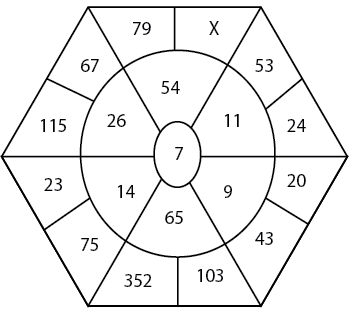Question 21

# Find the value of X.Solution

43 + 20 = 63, 63/7 = 9
352 + 103 = 455, 455/7 = 65
75 + 23 = 98, 98/7 = 14
115 + 67 = 182, 182/7 = 26
53 + 24 = 77, 77/7 = 11
$$\ \frac{\ 79+X}{7}=54$$
X = 299

• All Quant Formulas and shortcuts PDF
• 40+ previous papers with solutions PDF